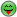# Help put equation into code

• 14 years ago

What I'm struggling with is understanding JPEG.

Now that noone is reading anymore, what I don't understand is the DCT.
Wikipedia shows it as (in Discrete cosine transform - Wikipedia, the free encyclopedia) (DCT-II):
Assuming N = 8, in doing one row (or column, but lets just assume row):

Xk = sum(0..7) { Xn * cos((PI/8)(n+.5)(k)) }

Now if X is a 8 element array of ints, and k and n are indeces, then:

Code:
`float sum = 0;for(int n=0;n<8;n++){sum += X[ n] * cos((PI/8)(n+.5)k);}`
I have no idea what 'k' is supposed to be. I think it's either 1..8, or it could be
1..7. It could be one of those ranges incrementing through the sum, or incrementing for each new Xk.

Can anyone help me out?

Many thanks in advance!

• 14 years ago

I managed to figure some things out. Xk is a different array from xn in the wiki formula.
Now for the 2-d version, I can take the Xk array and do the columns into another array.

This is indeed going to be slow, eh?

## Post a reply

### Enter your message below

Sign in or Join us (it's free).

## Quick links

### Contribute

Why not write for us? Or you could submit an event or a user group in your area. Alternatively just tell us what you think!

### Our tools

We've got automatic conversion tools to convert C# to VB.NET, VB.NET to C#. Also you can compress javascript and compress css and generate sql connection strings.

“The greatest performance improvement of all is when a system goes from not-working to working.”